# Sample on Math: Newton’s Method

Newton’s Method for Solving Nonlinear EquationsQuestion #1

Suppose a ball is dropped from a height of 2 meters onto a hard surface and the coefficient of restitution of the collision is .9. Write a script program to calculate the total distance the ball has traveled when it hits the surface for the n-th time.

Solution

Step 1. The coefficient of restitution for the case where the friction forces are negligible can be found as follows:

Cr=(h/H)1/2, where h is the bounce height and H is the drop height.

Since we know the initial height and the coefficient of restitution, we can obtain the bounce height, as follows:
h= Cr2*H.

Therefore, the total distance the ball has traveled when it hits the surface for the n-th time could be expressed with an equation:

h(n)=h(n-1)+2*h(n-1)* Cr2

Step 2. In a new document in MatLab, enter a script as shown in the figure 1 below: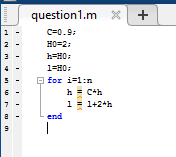Function 1

Step 3. In the command window, enter a number of bounces (n): >>n=10, for instance, and after that enter the name of the script. The result would be the total distance after each bounce.

Question #2

Modify the program from Question 1 to compute the total distance traveled by the ball while its bounces are at least 1 centimeter high. Use a while loop.

Solution

In order to solve Question #2, use the while loop, as shown in figure 1 below: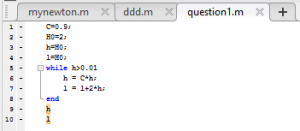Figure 1

The result, obtained after running the script, is shown below: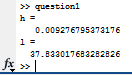Question #3

Perform the Bisection Method to solve a nonlinear equation until the error is bounded by a given tolerance. The given function is: f(x)=2×3+3x-1 with starting interval [0,1] and a tolerance of 10-8. How many steps does the program use to achieve this tolerance? How big is the final residual f(x)?

Solution

Step 1. A Bisection Method is a method which is used to solve non-linear equations where the initial bounds of argument are available (in or case this bound is [0,1] or a=0 and b=1). There are four input values in the program: f – inline function (f(x) in our case); a – left value of the initial bound; b – right bound of the initial area and tol – tolerance value (10-8 for this problem).

Step 2. A function body is shown in figure 1 below: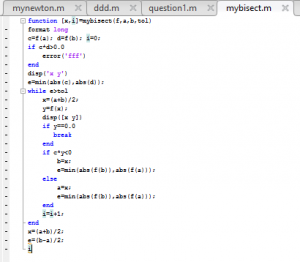Figure 1

After running this function, the next result was obtained: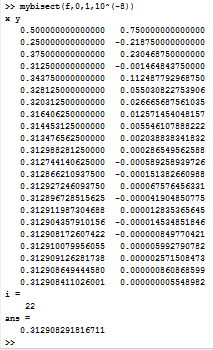Figure 2

Discussion: from the figure 2 above, the total amount of steps is i=22, the answer is 0.3129, and the final residual is f(x)=0.000000005548982.

The presented sample on math is a good example how to put your knowledge to use. Now, you can understand how to perform similar assignments yourself and what formulas to apply. If you feel you won’t be able to manage your math homework yourself,  our experts can help you no matter how tough your deadline is. Hurry up to get professional math assignment online assistance from AssignmentShark.